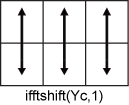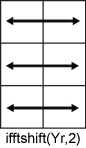# ifftshift

Inverse zero-frequency shift

## Syntax

``X = ifftshift(Y)``
``X = ifftshift(Y,dim)``

## Description

example

````X = ifftshift(Y)` rearranges a zero-frequency-shifted Fourier transform `Y` back to the original transform output. In other words, `ifftshift` undoes the result of `fftshift`.If `Y` is a vector, then `ifftshift` swaps the left and right halves of `Y`.If `Y` is a matrix, then `ifftshift` swaps the first quadrant of `Y` with the third, and the second quadrant with the fourth.If `Y` is a multidimensional array, then `ifftshift` swaps half-spaces of `Y` along each dimension.```

example

````X = ifftshift(Y,dim)` operates along the dimension `dim` of `Y`. For example, if `Y` is a matrix whose rows represent multiple 1-D transforms, then `ifftshift(Y,2)` swaps the halves of each row of `Y`.```

## Examples

collapse all

You can use the `fftshift` and `ifftshift` functions to swap left and right halves of a vector.

Create a vector containing an odd number of elements, and swap the left and right sides of the vector using the `fftshift` function.

```V = [1 2 3 4 5 6 7]; X = fftshift(V)```
```X = 1×7 5 6 7 1 2 3 4 ```

Use the `ifftshift` function to swap the left and right sides of `X`. The result is the same as the original vector `V`.

`Y = ifftshift(X)`
```Y = 1×7 1 2 3 4 5 6 7 ```

Calling the `fftshift` function twice does not necessarily reconstruct the original input.

`fftshift(fftshift(V))`
```ans = 1×7 2 3 4 5 6 7 1 ```

Rearrange the rows of a matrix to shift the nonnegative elements to the left.

```Y = [-2 -1 0 1 2; -10 -5 0 5 10]; X = ifftshift(Y,2)```
```X = 2×5 0 1 2 -2 -1 0 5 10 -10 -5 ```

## Input Arguments

collapse all

Input array, specified as a vector, a matrix, or a multidimensional array.

Data Types: `double` | `single` | `int8` | `int16` | `int32` | `int64` | `uint8` | `uint16` | `uint32` | `uint64` | `logical`
Complex Number Support: Yes

Dimension to operate along, specified as a positive integer scalar. If no value is specified, then `ifftshift` swaps along all dimensions.

• Consider an input matrix `Yc`. The operation `ifftshift(Yc,1)` swaps halves of each column of `Yc`.• Consider an input matrix `Yr`. The operation `ifftshift(Yr,2)` swaps halves of each row of `Yr`.Data Types: `double` | `single` | `int8` | `int16` | `int32` | `int64` | `uint8` | `uint16` | `uint32` | `uint64` | `logical`